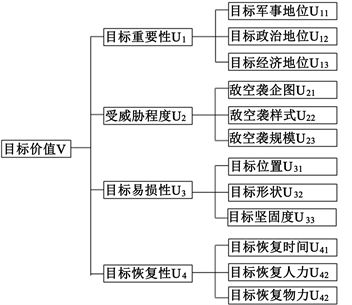# 基于Vague集的防空重点保卫目标优选与排序Selecting and Sequencing of the Important Defending Targets in Defense Zone Based on Vague Sets

• 全文下载: PDF(732KB)    PP.132-136   DOI: 10.12677/MSE.2019.82017
• 下载量: 237  浏览量: 308

Considering the deficiency of known air defense target value evaluation technology, a new method based on vague sets is presented. The indexes that affect evaluation of selecting and sequencing of the defending targets were analyzed; fuzzy value expression and weight calculation of the index is offered; this method can avoid subjective deviation resulted from expert evaluation method availa-bly. On this basis, a comprehensive evaluation model of the important defending targets in defense zone based on Vague Sets is established, and ranking method of the evaluation is given. Finally, via an example, the result of this evaluation technology is correct. The method proposed by this paper is valuable for the commander’s decision making.

1. 引言

2. Vague集基本理论

Gau和Buehrer早在1993年就提出了Vague集理论  ，由于Vague集理论比较符合人类思维活动，被广泛地推广应用。Vague集理论有以下定义：

3. 防空重点保卫目标综合评价指标体系

4. 基于Vague集的防空重点保卫目标优选与排序方法

4.1. 评价指标的模糊值表示Figure 1. Evaluation index system of important defending targets in defense zone

${t}_{i}{}_{j}=\left({x}_{i}{}_{j}-{x}_{j}^{\mathrm{min}}\right)/\left({x}_{j}^{\mathrm{max}}-{x}_{j}^{\mathrm{min}}\right)$ (1)

${f}_{i}{}_{j}=\left({x}_{j}^{\mathrm{max}}-{x}_{i}{}_{j}\right)/\left({x}_{j}^{\mathrm{max}}-{x}_{j}^{\mathrm{min}}\right)$ (2)

${t}_{i}{}_{j}=\left({x}_{j}^{\mathrm{max}}-{x}_{i}{}_{j}\right)/\left({x}_{j}^{\mathrm{max}}-{x}_{j}^{\mathrm{min}}\right)$ (3)

${f}_{i}{}_{j}=\left({x}_{i}{}_{j}-{x}_{j}^{\mathrm{min}}\right)/\left({x}_{j}^{\mathrm{max}}-{x}_{j}^{\mathrm{min}}\right)$ (4)

${F}_{ij}={t}_{i}{}_{j}+\left(1-{t}_{i}{}_{j}-{f}_{i}{}_{j}\right){t}_{i}{}_{j}/\left({t}_{i}{}_{j}+{f}_{i}{}_{j}\right)={t}_{i}{}_{j}/\left({t}_{i}{}_{j}+{f}_{i}{}_{j}\right)$ (5)

${t}_{ij}=0$ 时， ${F}_{ij}={t}_{i}{}_{j}+\left(1-{t}_{i}{}_{j}-{f}_{i}{}_{j}\right)\left(1-{f}_{i}{}_{j}\right)/2={\left(1-{f}_{i}{}_{j}\right)}^{2}/2$ (6)

${f}_{ij}=0$ 时， ${F}_{ij}={t}_{i}{}_{j}+\left(1-{t}_{i}{}_{j}-{f}_{i}{}_{j}\right)\left(1+{f}_{i}{}_{j}\right)/2={t}_{i}{}_{j}+\left(1-{f}_{i}{}_{j}\right)/2$ (7)

4.2. 评价指标权重的计算

$J\left({X}_{i},{X}^{*}\right)=\underset{j=1}{\overset{5}{\sum }}\sqrt{{f}_{ij}^{2}+{\left(1-{t}_{ij}\right)}^{2}}\cdot {w}_{j}$ (8)

$\begin{array}{l}min\text{\hspace{0.17em}}J\left(w\right)=\underset{i=1}{\overset{m}{\sum }}\underset{j=1}{\overset{5}{\sum }}\left[{f}_{ij}^{2}+{\left(1-{t}_{ij}\right)}^{2}\right]{w}_{j}^{2}\\ s.t.\text{ }{w}_{j}\ge 0,\text{ }\underset{j=1}{\overset{5}{\sum }}{w}_{j}=1\end{array}$ (9)

${w}_{j}=\frac{1/\underset{j=1}{\overset{5}{\sum }}\frac{1}{\underset{i=1}{\overset{m}{\sum }}\left[{f}_{ij}^{2}+{\left(1-{t}_{ij}\right)}^{2}\right]}}{\underset{i=1}{\overset{m}{\sum }}\left[{f}_{ij}^{2}+{\left(1-{t}_{ij}\right)}^{2}\right]},\text{ }j=1,2,3,4$ (10)

4.3. 基于Vague集的防空重点保卫目标优选方法

1) 构造模糊值矩阵 $F={\left({F}_{ij}\right)}_{m×n}$

2) 计算各指标权重。

3) 分别确定方案集的最优、最劣点 ${X}^{+}、{X}^{-}$

4) 计算每个方案到最优点 ${X}^{+}$ 的接近度 ${E}_{i}$

${d}_{i}^{+}=d\left({X}_{i},{X}^{+}\right)=\underset{j=1}{\overset{4}{\sum }}\sqrt{{\left({f}_{ij}-{f}_{{p}_{j}}\right)}^{2}+{\left({t}_{ij}-{t}_{{p}_{j}}\right)}^{2}}\cdot {w}_{j},\text{ }i=1,2,\cdots ,m$ (11)

${d}_{i}^{-}=d\left({X}_{i},{X}^{-}\right)=\underset{j=1}{\overset{4}{\sum }}\sqrt{{\left({f}_{ij}-{f}_{{q}_{j}}\right)}^{2}+{\left({t}_{ij}-{t}_{{q}_{j}}\right)}^{2}}\cdot {w}_{j},\text{ }i=1,2,\cdots ,m$ (12)

${E}_{i}={d}_{i}^{-}/\left({d}_{i}^{-}+{d}_{i}^{+}\right),\text{ }i=1,2,\cdots ,m$ (13)

5) 通过 ${E}_{i}$ 值对待评价的防空重点保卫目标进行优选与排序。

5. 实例分析Table 1. Evaluation indexes of important defending targets in defense zone

1) 评价指标模糊值表示

${X}_{1}=\left\{\left({U}_{1},\text{\hspace{0.17em}}\left[0.00,0.00\right]\right),\text{\hspace{0.17em}}\left({U}_{2},\text{\hspace{0.17em}}\left[1.00,1.00\right]\right),\text{\hspace{0.17em}}\left({U}_{3},\text{\hspace{0.17em}}\left[0.14,0.14\right]\right),\text{\hspace{0.17em}}\left({U}_{4},\text{\hspace{0.17em}}\left[1.00,1.00\right]\right)\right\}$

${X}_{2}=\left\{\left({U}_{1},\text{\hspace{0.17em}}\left[1.00,1.00\right]\right),\text{\hspace{0.17em}}\left({U}_{2},\text{\hspace{0.17em}}\left[0.19,0.19\right]\right),\text{\hspace{0.17em}}\left({U}_{3},\text{\hspace{0.17em}}\left[1.00,1.00\right]\right),\text{\hspace{0.17em}}\left({U}_{4},\text{\hspace{0.17em}}\left[0.00,0.00\right]\right)\right\}$

${X}_{3}=\left\{\left({U}_{1},\text{\hspace{0.17em}}\left[0.83,0.83\right]\right),\text{\hspace{0.17em}}\left({U}_{2},\text{\hspace{0.17em}}\left[0.00,0.00\right]\right),\text{\hspace{0.17em}}\left({U}_{3},\text{\hspace{0.17em}}\left[0.46,0.46\right]\right),\text{\hspace{0.17em}}\left({U}_{4},\text{\hspace{0.17em}}\left[0.18,0.18\right]\right)\right\}$

${X}_{4}=\left\{\left({U}_{1},\text{\hspace{0.17em}}\left[0.79,0.79\right]\right),\text{\hspace{0.17em}}\left({U}_{2},\text{\hspace{0.17em}}\left[0.35,0.35\right]\right),\text{\hspace{0.17em}}\left({U}_{3},\text{\hspace{0.17em}}\left[0.00,0.00\right]\right),\text{\hspace{0.17em}}\left({U}_{4},\text{\hspace{0.17em}}\left[0.02,0.02\right]\right)\right\}$

2) 计算各指标权重分别为： ${w}_{1}=0.4078$${w}_{2}=0.2106$${w}_{3}=0.2153$${w}_{4}=0.1663$

3) 确定方案集的最优、最劣点 ${X}^{+}、{X}^{-}$

${X}^{+}=\left\{\left({U}_{1},\text{\hspace{0.17em}}\left[1.00,1.00\right]\right),\text{\hspace{0.17em}}\left({U}_{2},\text{\hspace{0.17em}}\left[1.00,1.00\right]\right),\text{\hspace{0.17em}}\left({U}_{3},\text{\hspace{0.17em}}\left[1.00,1.00\right]\right),\text{\hspace{0.17em}}\left({U}_{4},\text{\hspace{0.17em}}\left[1.00,1.00\right]\right)\right\}$

${X}^{-}=\left\{\left({U}_{1},\text{\hspace{0.17em}}\left[0.00,0.00\right]\right),\text{\hspace{0.17em}}\left({U}_{2},\text{\hspace{0.17em}}\left[0.00,0.00\right]\right),\text{\hspace{0.17em}}\left({U}_{3},\text{\hspace{0.17em}}\left[0.00,0.00\right]\right),\text{\hspace{0.17em}}\left({U}_{4},\text{\hspace{0.17em}}\left[0.00,0.00\right]\right)\right\}$

4) 确定各方案 ${X}_{i}$ 到最优、最劣点的距离 ${d}_{i}^{+}、{d}_{i}^{-}$

${d}_{1}^{+}=0.7953$${d}_{2}^{+}=0.4807$${d}_{3}^{+}=0.7607$${d}_{4}^{+}=0.8496$

${d}_{1}^{-}=0.5756$${d}_{2}^{-}=0.9377$${d}_{3}^{-}=0.6611$${d}_{4}^{-}=0.5645$

5) 确定各方案 ${X}_{i}$ 到最优点 ${X}^{+}$ 的接近度 ${E}_{i}$

${E}_{1}=0.4199$${E}_{2}=0.6611$${E}_{3}=0.4650$${E}_{4}=0.3992$

6. 结束语

  张海兵, 徐诚. 基于改进AHP 的目标价值评估模型分析[J]. 弹箭与制导学报, 2007, 27(1): 228-230.  郭积宁, 沈军, 潘丽萍. 空军机场地面目标威胁评估方法[J]. 桂林空军学院学报, 2011, 28(6): 20-23.  刘琼, 艾云平, 王卓柱, 等. 多层次灰色聚类决策对目标价值排序的应用[J]. 火力与指挥控制, 2008, 33(5): 79-81.  孔健, 郝强, 王艳正, 等. 大区域防空作战重点保卫目标的优选与排序[J]. 现代防御技术, 2007, 35(3): 68-71.  Gau, W.L. and Buehrer, D.J. (1993) Vague Sets. IEEE Transactions on Systems, Man, and Cybernetics, 23, 610-614. https://doi.org/10.1109/21.229476  周晓光, 谭春桥, 张强. 基于Vague集的决策理论与方法[M]. 北京: 科学出版社, 2009.  宁伟华, 李海龙, 席吉虎. 基于不确定多属性决策的防空重点保卫目标优选与排序[J]. 空军工程大学学报(自然科学版), 2006, 7(4): 28-31.  彭方明, 邢清华, 王三涛. 基于Vague集TOPSIS法的空中目标威胁评估[J]. 电光与控制, 2010, 17(10): 23-27.  要瑞璞, 沈惠璋. 不确定多属性Vague集决策方法[J]. 计算机工程与应用, 2010, 46(14): 48-49